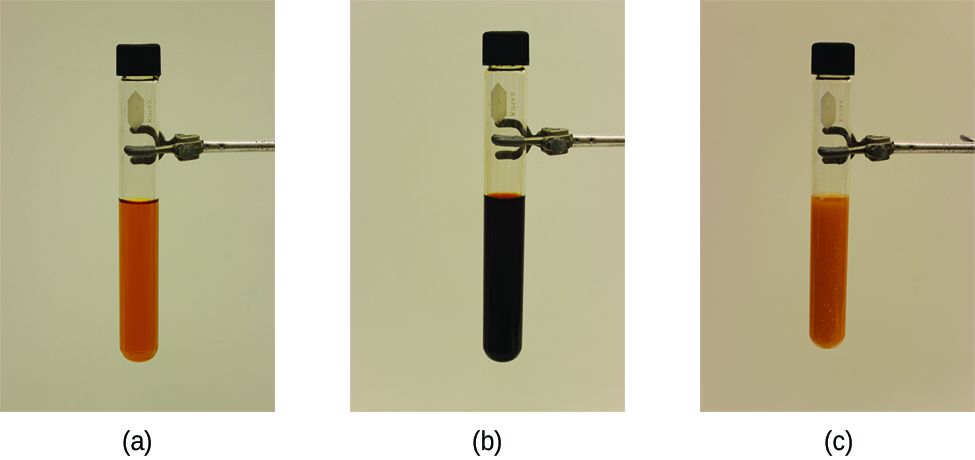# 13.3 Shifting equilibria: le châtelier’s principle

 Page 1 / 10
By the end of this section, you will be able to:
• Describe the ways in which an equilibrium system can be stressed
• Predict the response of a stressed equilibrium using Le Châtelier’s principle

As we saw in the previous section, reactions proceed in both directions (reactants go to products and products go to reactants). We can tell a reaction is at equilibrium if the reaction quotient ( Q ) is equal to the equilibrium constant ( K ). We next address what happens when a system at equilibrium is disturbed so that Q is no longer equal to K . If a system at equilibrium is subjected to a perturbance or stress    (such as a change in concentration) the position of equilibrium    changes. Since this stress affects the concentrations of the reactants and the products, the value of Q will no longer equal the value of K . To re-establish equilibrium, the system will either shift toward the products (if Q< K) or the reactants (if Q> K ) until Q returns to the same value as K .

This process is described by Le Châtelier's principle    : When a chemical system at equilibrium is disturbed, it returns to equilibrium by counteracting the disturbance. As described in the previous paragraph, the disturbance causes a change in Q ; the reaction will shift to re-establish Q = K .

## Predicting the direction of a reversible reaction

Le Châtelier's principle can be used to predict changes in equilibrium concentrations when a system that is at equilibrium is subjected to a stress. However, if we have a mixture of reactants and products that have not yet reached equilibrium, the changes necessary to reach equilibrium may not be so obvious. In such a case, we can compare the values of Q and K for the system to predict the changes.

## Effect of change in concentration on equilibrium

A chemical system at equilibrium can be temporarily shifted out of equilibrium by adding or removing one or more of the reactants or products. The concentrations of both reactants and products then undergo additional changes to return the system to equilibrium.

The stress on the system in [link] is the reduction of the equilibrium concentration of SCN (lowering the concentration of one of the reactants would cause Q to be larger than K ). As a consequence, Le Châtelier's principle leads us to predict that the concentration of Fe(SCN) 2+ should decrease, increasing the concentration of SCN part way back to its original concentration, and increasing the concentration of Fe 3+ above its initial equilibrium concentration.(a) The test tube contains 0.1 M Fe 3+ . (b) Thiocyanate ion has been added to solution in (a), forming the red Fe(SCN) 2+ ion. Fe 3+ ( a q ) + SCN − ( a q ) ⇌ Fe ( SCN ) 2+ ( a q ) . (c) Silver nitrate has been added to the solution in (b), precipitating some of the SCN − as the white solid AgSCN. Ag + ( a q ) + SCN − ( a q ) ⇌ AgSCN ( s ) . The decrease in the SCN − concentration shifts the first equilibrium in the solution to the left, decreasing the concentration (and lightening color) of the Fe(SCN) 2+ . (credit: modification of work by Mark Ott)

The effect of a change in concentration on a system at equilibrium is illustrated further by the equilibrium of this chemical reaction:

#### Questions & Answers

What is whizatron?
Frendick Reply
What is stoichometry
ngwuebo Reply
what is atom
yinka Reply
An indivisible part of an element
ngwuebo
the smallest particle of an element which is indivisible is called an atom
Aloaye
An atom is the smallest indivisible particle of an element that can take part in chemical reaction
Alieu
is carbonates soluble
Ebuka Reply
what is the difference between light and electricity
Joshua Reply
What is atom? atom can be defined as the smallest particles
Adazion
what is the difference between Anode and nodes?
Adazion
What's the net equations for the three steps of dissociation of phosphoric acid?
Lisa Reply
what is chemistry
Prince Reply
the study of matter
Reginald
what did the first law of thermodynamics say
Starr Reply
energy can neither be created or distroyed it can only be transferred or converted from one form to another
Adedeji
Graham's law of Diffusion
Ayo Reply
what is melting vaporization
Anieke Reply
melting and boiling point explain in term of molecular motion and Brownian movement
Anieke
Scientific notation for 150.9433962
Steve Reply
what is aromaticity
Usman Reply
aromaticity is a conjugated pi system specific to organic rings like benzene, which have an odd number of electron pairs within the system that allows for exceptional molecular stability
Pookieman
what is caustic soda
Ogbonna Reply
sodium hydroxide (NaOH)
Kamaluddeen
what is distilled water
Rihanat
is simply means a condensed water vapour
Kamaluddeen
advantage and disadvantage of water to human and industry
Abdulrahman Reply
a hydrocarbon contains 7.7 percent by mass of hydrogen and 92.3 percent by mass of carbon
Timothy Reply

### Read also:

#### Get the best Chemistry course in your pocket!

Source:  OpenStax, Chemistry. OpenStax CNX. May 20, 2015 Download for free at http://legacy.cnx.org/content/col11760/1.9
Google Play and the Google Play logo are trademarks of Google Inc.

Notification Switch

Would you like to follow the 'Chemistry' conversation and receive update notifications?ByByByByBy